# Duality

(diff) ← Older revision | Latest revision (diff) | Newer revision → (diff)

Duality in algebraic geometry. Duality between the different cohomology spaces on algebraic varieties.

Cohomology of coherent sheaves. Letbe a non-singular algebraic variety of dimensionover an algebraically closed fieldand letbe a locally free sheaf on. Serre's duality theorem states that the finite-dimensional cohomology (vector) spaces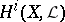andare mutually dual. Here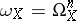is the sheaf of germs of regular differential forms of degreeon, and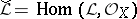is the locally free sheaf dual to. If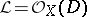is the invertible sheaf corresponding to a divisoron, this theorem establishes the equality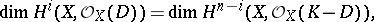whereis the canonical divisor on. If, a relation equivalent to the above was found as early as the 19th century. There exists a generalization of Serre's theorem to the case of cohomology of arbitrary coherent sheaves on complete algebraic varieties , . In particular, if the varietyis a Cohen–Macaulay subvariety (e.g. a locally complete intersection) of codimensionin a non-singular projective variety, there is duality between the-space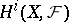and the space of global Ext's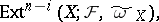where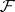is a coherent sheaf on,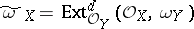(Grothendieck's dualizing sheaf), while. Here, the sheaf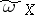is invertible if and only ifis a Gorenstein scheme (cf. Gorenstein ring).

Etale cohomology. Letbe a complete connected non-singular algebraic variety of dimensionover an algebraically closed field; letbe an integer which is relatively prime to the characteristic of the field; letbe a locally free (in the étale topology) sheaf of-modules on; and let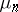be the sheaf of-th power roots of unity. Then there exists a non-degenerate pairing of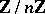-modules :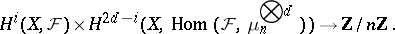A more general duality theorem concerns smooth, but not necessarily complete, varieties . There exists a non-degenerate pairing of-moduleswhere cohomology with compact support is found on the left-hand side. If the fieldis the algebraic closure of a field,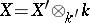and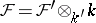, then the Galois group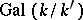acts onand the preceding pairing is a pairing of-modules.

Poincaré's duality theorem is an analogue of the first of the theorems given for-adic cohomology: There exists a non-degenerate pairing of-moduleswhere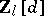is the Tate sheaf, which is non-canonically isomorphic to the sheaf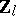(cf.-adic cohomology). Hence the isomorphism of-spaces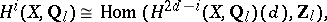and, in particular, the equality of the Betti numbers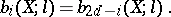As in the case of cohomology of coherent sheaves, these results can be generalized to the relative case of a proper scheme morphism, formulated in the language of derived categories .

Other cohomology theories. Analogues of Poincaré's theorem are valid in the theory of crystalline cohomology , and de Rham cohomology over a field of characteristic zero . In number-theoretic applications cohomology of sheaves on the flat Grothendieck topology of arithmetic schemes are important. Duality theorems  are applicable to special cases of such cohomology theories.

## Contents

How to Cite This Entry:
Duality. Encyclopedia of Mathematics. URL: http://encyclopediaofmath.org/index.php?title=Duality&oldid=18022
This article was adapted from an original article by I.V. Dolgachev (originator), which appeared in Encyclopedia of Mathematics - ISBN 1402006098. See original article